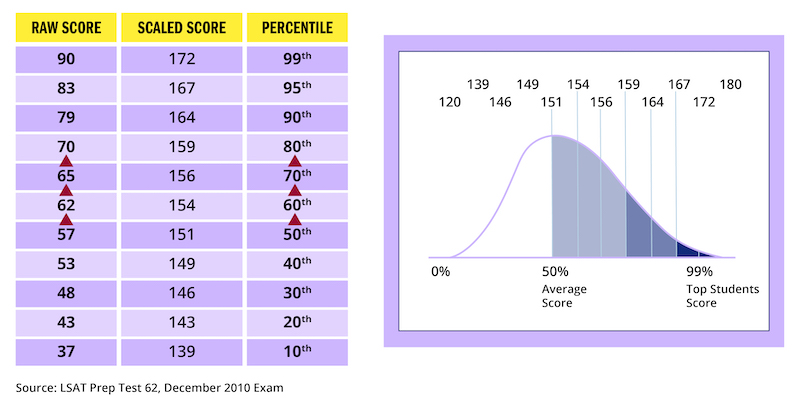# LSAT Raw Score Conversion

Find out what a raw, scaled and percentile LSAT score is and how to convert that one score into another.

## LSAT Score Types

A raw LSAT score is simply the number of questions answered correctly.

A scaled LSAT score is a conversion of the raw score, also known as the familiar 120–180 number. For example, a raw score of 67 is 67 correct answers, which converts to a scaled score of 156. A raw score of 58—meaning 58 correct answers—converts to a scaled score of 151.

On a different test, a raw score of 58 might convert to a 150 or a 152. To account for differences in overall difficulty, each iteration of the test has a slightly different raw score-to-scaled score conversion table.

LSAT percentile scores indicate how a test taker performed relative to other test takers over a three-year period. The conversion from scaled score to percentile score remains relatively stable, with only minor variations over the years. Test after test, a 151 scaled score is approximately a 50th percentile score.

## LSAT Score Conversion Chart

LSAT scoring looks a bit different these days, as the exam only has three scored sections and roughly 75 points. Below is the scoring scale LSAC released for their May 2020 LSAT Flex. Even though the LSAT Flex is no longer administered, its scoring is scale will remain relevant to the new LSAT going forward, as it also contained three scored sections.

## Historic LSAT Score Conversion Charts

Take a look at an example conversion chart based on PrepTest 74:

These historic examples (PrepTests 1-80) feature raw score conversions from when there were 4 scored sections and roughly 100 points. The new LSAT only has three scored sections and roughly 75 points.

Take a look at an example conversion chart with scoring ranges based on PrepTest 62:The way in which the LSAT is scored has three important implications for your performance:

1. The number of right answers determines your score. There is no guessing penalty. Never leave a question blank on the LSAT.
2. Every question is worth the same, regardless of how hard it is. Learn to spot difficult questions and leave them for the end of each section. Find the easy questions and rack up points. If you’re going to run out of time or need to guess, you want to do so on the tough stuff.